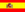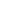home | más chusmeríos | otros temas de Física | lecciones del maestro Ciruela | tonterías | @NO ME SALEN
THEORETICAL NOTES AND EXCERCISES OF PHYSICS AND BIOPHYSICSMiscellany
Passage units
EQUIVALENCES

It consists in replacing the units you don´t want to appear, by their equivalents, expressed in the units you want to have.

 Example 1) convert 20 m/s to km/h As one kilometer (kilo means a thousand) are 1,000 meters, 1 meter is the thousandth of a kilometer; In an equation: 1 m = 0.001 km (keep this equation in your heart) As one hour equals to 3,600 seconds (even my seven year old daughter know this), one second is equal to one 3,600th of an hour; In an equation: 1 s = 0.0002778 h (keep this one in your liver) Now, rewrite the speed you want to convert (20 m/s), but, this time, where it says m, write it´s equal according to the equation you hold dearly in your heart, and where it says s, write it´s equal according to the equation you hold in your liver. Then, you have this: 20 . 0.001 km / 0.0002778 h Take a look, you have three numbers and two units. The units are the ones that you want, and for the numbers, you can ask the calculator to merge them into one. This is: 20 m/s = 72 km/h Example 2) convert 3.500 km/h² to m/s² As one kilometer (kilo means a thousand) are 1,000 meters 1 km = 1,000 m (an equivalency) As one hour equals to 3,600 seconds, in one hour times one hour (h2) there are 3,600 seconds times 3,600 seconds. 1h² = 12,960,000 s² (another equivalency) Now rewrite the speed you want to convert (35 km/h2) but, where it says km, write it´s equivalent, and where it says h2 write the second equivalency. Then, you have this: 3,500 . 1,000 m / 12,960,000 s² Take a look, you have three numbers and two units. The units are the ones that you want, and for the numbers... 3,500 km/h² = 0.27 m/s² Example 3) convert 0,7 kgf/cm to N/m Every mortal knows that 1 kgf = 10 N (an equivalency) It is also true that 1 cm = 0,01 m we replace, and... 0,7 kgf/cm = 0,7 . 10 N / 0,01 m = 700 N/m Example 4) convert 60.000  J/day to W 1 W (1 Watt) is, by definition, 1 J/s. 1 J = 1 W . s And in one day there are like 86.400 seconds; replacing the equivalences... 60.000  J/day = 600  1 W.s /86.400 s 60.000  J/day = 0,694  1 WSome rights reserved. Reproduction allowe by quoting the source. Translated by Santi Pisci. Last Updated jul-15. Buenos Aires, Argentina.